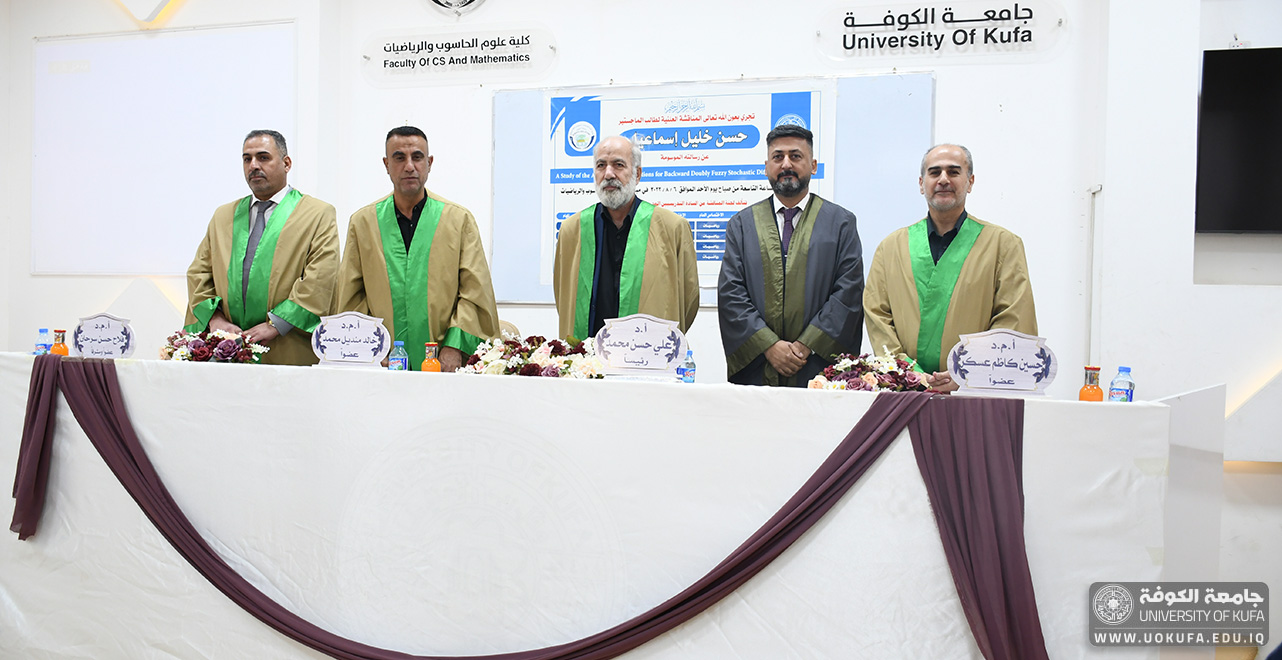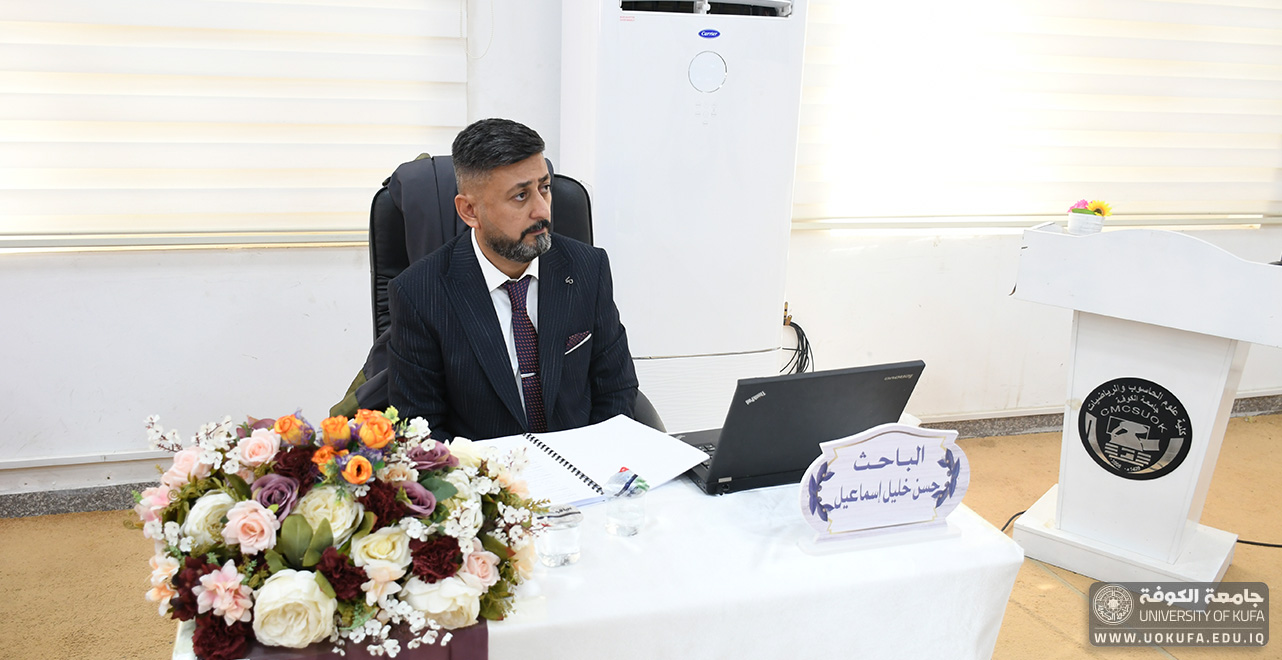Home / Scientific activities / Articles / Master’s thesis at the faculty of Computer Science and Mathematics discussed (A study of approximation solutions of backward doubly fuzzy stochastic differential equations)# Master’s thesis at the faculty of Computer Science and Mathematics discussed (A study of approximation solutions of backward doubly fuzzy stochastic differential equations)

### The Faculty of Computer Science and Mathematics, Department of Mathematics, discussed the master’s thesis entitled (A study of approximation solutions of backward doubly fuzzy stochastic differential equations) for a master’s student (Hassan Khalil Ismail) and under the supervision of (Asst. Prof. Dr. Falah Hassan Sarhan). This thesis presented a study on backward doubly fuzzy stochastic differential equations, where in the first part of this work the researcher studied the convergence of the backward fuzzy stochastic differential equations and discussed the existence and uniqueness of the approximate solutions to the equation under Lipschitz conditions and using the method of the mean square error between the general solution and the approximate solution of the above equation. In the second part he demonstrated the convergence of the backward doubly fuzzy stochastic differential equations under the same conditions and in the same way also discussed the existence and uniqueness of approximate solutions to the aforementioned equations. Finally the thesis is successfully accepted.## CSM Department at Kufa University Cooperates ,with an International Vision, to Fight Against Drugs.

To promote health awareness and fight against drugs the Faculty of Computer Science and Mathematics …